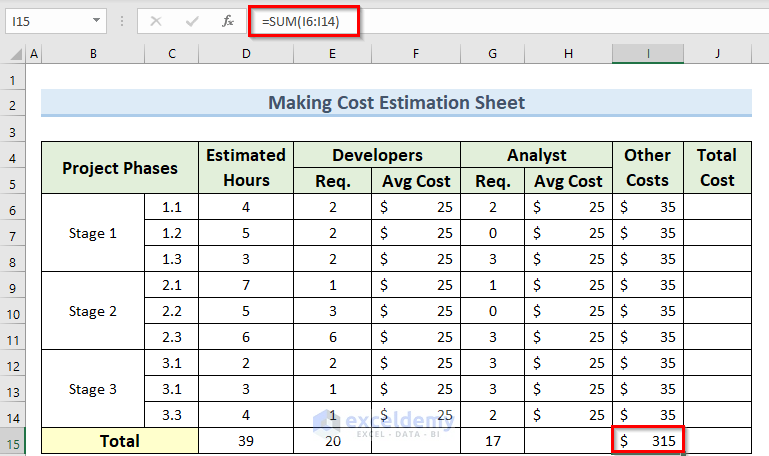# How to Make Cost Estimation Sheet in Excel (with Easy Steps)

Get FREE Advanced Excel Exercises with Solutions!

In this tutorial, I am going to show you the step-by-step procedures to make a cost estimation sheet in Excel. You can quickly use these methods even in large datasets to generate simple excel workbooks for estimating project costs. Throughout this tutorial, you will also learn some important excel tools and functions which will be very useful in any excel related task.

## Step-by-Step Procedures to Make Cost Estimation Sheet in Excel

We have taken a concise dataset to explain the steps clearly. The dataset has approximately 11 rows and columns. Initially, we are keeping all the cells in General format and the monetary values in Accounting format. For all the datasets, we have 6 unique columns which are Project Stages, Estimated Hours, Developers, Analyst, Other Costs, and Total Cost. However, we may vary the number of columns later on if that is needed.

### Step-1: Creating Base Excel Data Sheet for Cost Estimation

• First, create a starting empty dataset as in the image below.### Step-2: Inserting Necessary Data Values

• To begin with, insert the necessary values for the Estimated Hours, Developers, Analyst, Other Costs, and Total Cost columns properly.• Next, go to cell D15 and type in the following formula:
`=SUM(D6:D14)`• Now, navigate to cell E15 and enter the formula below:
`=SUM(E6:E14)`
• Then, press Enter and similarly type this formula in cell G15.### Step-3: Calculating Total Cost

• To start with, go to cell I15 and insert this formula:
`=SUM(I6:I14)`
• After that press the Enter key to confirm this formula.

###• Now, type in the following formula in cell J6:
`=(D6*E6*F6)+(D6*G6*H6)`
• Then, press Enter and copy this formula down using Fill Handle.• Next, navigate to cell J15 and type the following formula:
`=SUM(J6:J14)`
• Finally, press the Enter key and this will find out the final total cost.So by following the above steps we can easily create a detailed cost estimation sheet in Excel.

## Creating Cost Estimation Sheet for Building Construction in Excel

Now, we will see how we can quickly generate a cost estimation sheet for a building construction project.

Steps:

• To begin with, create a basic dataset including the columns Particular, Quantity, Rate, and Amount as in the image below.• Next, go to cell E5 and type in the formula below:
`=C5*D5`
• Now, press Enter and copy this formula down to cell E9.• After that insert the following formula in cell E10 and press Enter:
`=SUM(E5:E9)`• Now, create a Bill of Quantity dataset as in the image below.• Then, go to cell F5 and insert the following formula:
`=D5*E5`
• Next, press Enter to confirm and copy this formula to cell F7 using Fill Handle.• Then, enter the formula below in cell F8 and press Enter to confirm:
`=SUM(F5:F7)`• After that, go to cell F9 and type in the following formula:
`=D9*E9`
• Now, press Enter to confirm this formula and take this formula to the other cells by dragging the Fill Handle.• Now, go to cell F12 and insert the below formula:
`=SUM(F9:F11)`
• Immediately, you should get the value of the Total Labor Cost.• Then, type in the following formula in cell F13:
`=F8+F12`
• Now, press the Enter key on the keyboard and this should evaluate the Total Material & Labor Cost.• Next, create a simple Summary data table as in the image below.• Now, go to cell C5 and insert the following formula:
`='Bill of Quantity'!F8`
• Then, confirm this formula by pressing Enter key on the keyboard.• After that, write the formula below in cell C6:
`='Bill of Quantity'!F12`
• Now, press Enter again to confirm this formula and get the Labor Cost value in cell C6.• Next, insert this last formula in cell C8:
`=SUM(C5:C7)`
• Finally, press Enter to get the final Grand Total value of the project.So, in this way, we can quickly create a cost estimation sheet for building construction or similar projects.

## Conclusion

I hope that you were able to apply the methods that I showed in this tutorial on how to make a cost estimation sheet in Excel. As you can see, there are quite a few ways to achieve this. So wisely choose the method that suits your situation best. If you get stuck in any of the steps, I recommend going through them a few times to clear up any confusion. Lastly, to learn more excel techniques, follow our ExcelDemy website. If you have any queries, please let me know in the comments.

## Related ArticlesNazmul Hossain Shovon

Hello, I am Nazmul Hossain. I am currently working full-time in Exceldemy as an Excel & VBA Content Developer. I have completed my bachelors in Naval Architecture and Marine Engineering from Bangladesh University of Engineering and Technology. I am interested in working with MS Excel. I also like coding web applications a lot.

We will be happy to hear your thoughtsAdvanced Excel Exercises with Solutions PDF